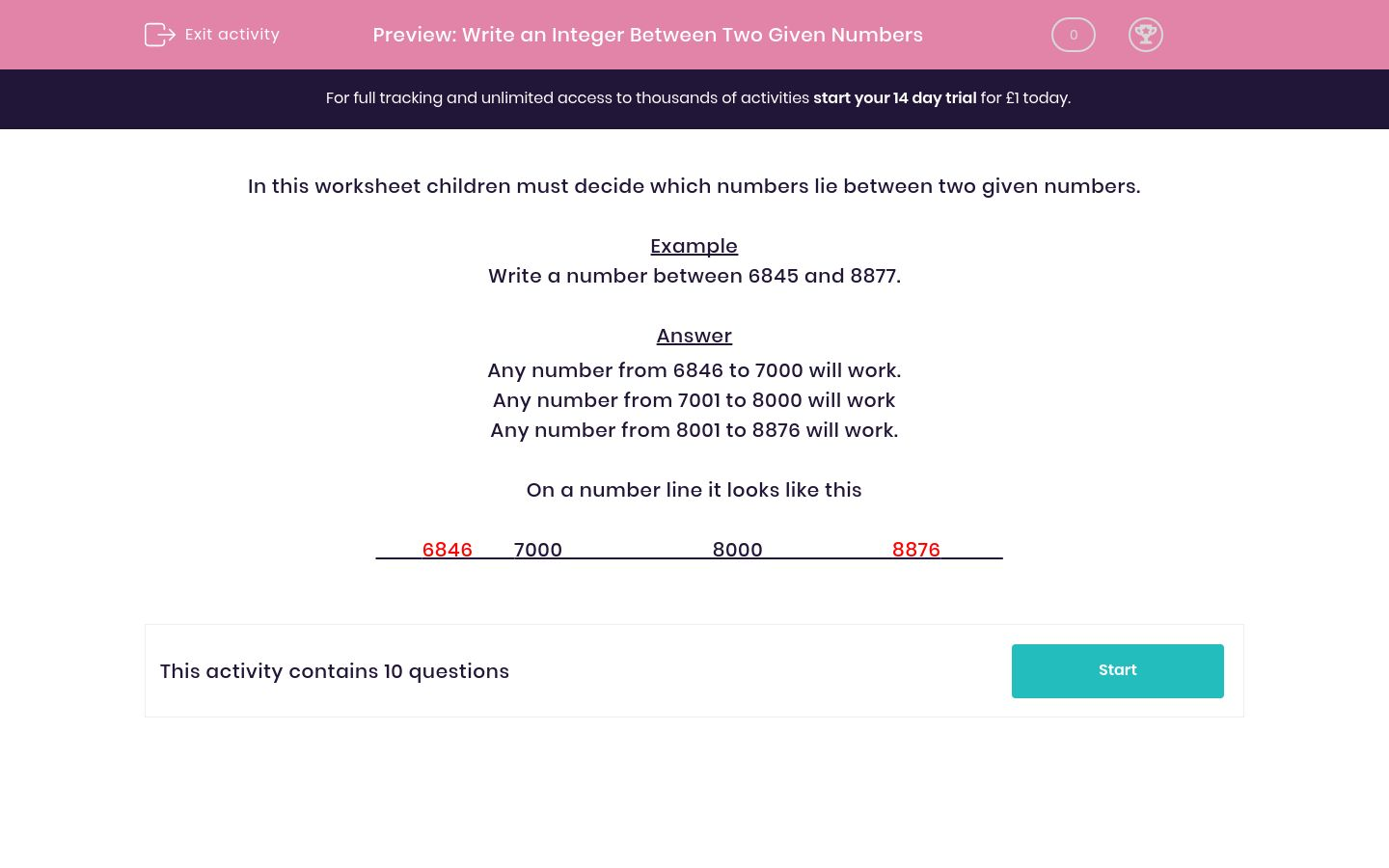# Measuring Shapes: Calculating Area (2)

In this worksheet, students calculate the areas of rectangles in cm².Key stage:  KS 2

Curriculum topic:   Maths and Numerical Reasoning

Curriculum subtopic:   Perimeter and Area

Difficulty level:### QUESTION 1 of 10

In this worksheet, you must find the areas of rectangles in cm².

Example

Find the area of the rectangle.
Multiply the length by the width.11 cm
12 cm
cm2

We multiply together the length by the width.

12cm × 11cm = 132cm²

We just write 132 in the answer box.

Find the area of the rectangle.
Multiply the length by the width.12 cm
8 cm
cm2

Find the area of the rectangle.
Multiply the length by the width.14 cm
10 cm
cm2

Find the area of the rectangle.
Multiply the length by the width.7 cm
10 cm
cm2

Find the area of the rectangle.
Multiply the length by the width.9 cm
12 cm
cm2

Find the area of the rectangle.
Multiply the length by the width.9 cm
14 cm
cm2

Find the area of the rectangle.
Multiply the length by the width.9 cm
8 cm
cm2

Find the area of the rectangle.
Multiply the length by the width.9 cm
11 cm
cm2

Find the area of the rectangle.
Multiply the length by the width.14 cm
13 cm
cm2

Find the area of the rectangle.
Multiply the length by the width.11 cm
13 cm
cm2

Find the area of the rectangle.
Multiply the length by the width.9 cm
13 cm
cm2
• Question 1

Find the area of the rectangle.
Multiply the length by the width.12 cm
8 cm
cm2
96
EDDIE SAYS
12cm x 8cm = 96cm
• Question 2

Find the area of the rectangle.
Multiply the length by the width.14 cm
10 cm
cm2
140
EDDIE SAYS
14cm x 10cm = 140cm
• Question 3

Find the area of the rectangle.
Multiply the length by the width.7 cm
10 cm
cm2
70
EDDIE SAYS
10cm x 7cm = 70cm
• Question 4

Find the area of the rectangle.
Multiply the length by the width.9 cm
12 cm
cm2
108
EDDIE SAYS
12cm x 9cm = 108cm
• Question 5

Find the area of the rectangle.
Multiply the length by the width.9 cm
14 cm
cm2
126
EDDIE SAYS
9cm x 14cm = 126cm
• Question 6

Find the area of the rectangle.
Multiply the length by the width.9 cm
8 cm
cm2
72
EDDIE SAYS
8cm x 9cm = 72cm
• Question 7

Find the area of the rectangle.
Multiply the length by the width.9 cm
11 cm
cm2
99
EDDIE SAYS
11cm x 9cm = 99cm
• Question 8

Find the area of the rectangle.
Multiply the length by the width.14 cm
13 cm
cm2
182
EDDIE SAYS
14cm x 13cm = 182cm
• Question 9

Find the area of the rectangle.
Multiply the length by the width.11 cm
13 cm
cm2
143
EDDIE SAYS
11cm x 13cm = 143cm
• Question 10

Find the area of the rectangle.
Multiply the length by the width.9 cm
13 cm
cm2
117
EDDIE SAYS
13cm x 9cm = 117cm
---- OR ----

Sign up for a £1 trial so you can track and measure your child's progress on this activity.

### What is EdPlace?

We're your National Curriculum aligned online education content provider helping each child succeed in English, maths and science from year 1 to GCSE. With an EdPlace account you’ll be able to track and measure progress, helping each child achieve their best. We build confidence and attainment by personalising each child’s learning at a level that suits them.

Get started